Next: 3.6 Analysis of Closed Hashing Up: 3.5 Hashing Functions Previous: 3.5.2 Multiplication Method

## 3.5.3 Universal Hashing

This involves choosing a hash function randomly in a way that is independent of the keys that are actually going to be stored. We select the hash function at random from a carefully designed class of functions.
• Let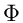be a finite collection of hash functions that map a given universe U of keys into the range {0, 1, 2,..., m - 1}.
•is called universal if for each pair of distinct keys x, y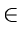U, the number of hash functions hfor which h(x) = h(y) is precisely equal to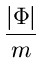• With a function randomly chosen from, the chance of a collision between x and y where x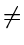y is exactly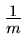.

Example of a universal class of hash functions:

Let table size m be prime. Decompose a key x into r +1 bytes. (i.e., characters or fixed-width binary strings). Thus

x = (x0, x1,..., xr)

Assume that the maximum value of a byte to be less than m.

Let a = (a0, a1,..., ar) denote a sequence of r + 1 elements chosen randomly from the set {0, 1,..., m - 1}. Define a hash function haby

ha(x) =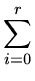aixi mod m

With this definition,=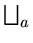{ha} can be shown to be universal. Note that it has mr + 1 members.Next: 3.6 Analysis of Closed Hashing Up: 3.5 Hashing Functions Previous: 3.5.2 Multiplication Method
eEL,CSA_Dept,IISc,Bangalore